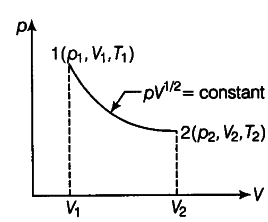# Consider a p-V diagram in which the path followed

Consider a p-V diagram in which the path followed by one mole of perfect gas in a cylindrical container is shown.(i) Find the work done when the gas is taken from state 1 to state 2.
(ii) What is the ratio of temperatures { T }_{ 1 } /
{ T }_{ 2 }, if
{ V }_{ 2 }=
{ 2V }_{ 1 }
(iii) Given the internal energy for one mole of gas at temperature T is (3/2) RT, find the heat supplied to the gas when it is taken from states 1 to 2, with { V }_{ 2 } =
{ 2V }_{ 1 } .